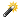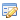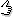### How do I calculate the bearing of a line?

Occurrence

The MapInfo Ruler tool does not give a bearing angle!
How do I calculate the bearing of a line, or between two points?

Solution

To calculate a bearing interactively with a ruler tool, use Discover's
QUERY>Map Object>Location Info

Or to calculate a selected lines bearing and saved in as a attribute use -
QUERY>Map Object>Line Orientation

MapInfo MapCAD, which is now turned on by default, adds a tool under -
SPATIAL>Calculate>Calculate Direction

This is incorrect. It calculates a mathematical standard cartesian angle, which is counter clockwise from the right hand direction (East).

To convert this into a GIS bearing, which is clockwise from North (top of map) you need to apply the following -

degResult = 90.0 - mathBasedDeg;
if (degResult < 0)
degResult += 360;
if (degResult > 360)
degResult -= 360;

### Would you like to...Print this pageEmail this pagePost a commentSubscribe meRemove HighlightingEdit this article

100%0%